Array out of Range Error while using [i+2] in getting Moving Average value in MQL5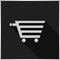22

Hello guys;

I have an issue, I am trying to code an Arrow indicator for Moving Average crossover, but I always encounter an "Array out of Range Error" on any line with [i+2], and when i cooment out this lines, I later have the same "Array out of Range Error" on any line with [i+1] && [i+3]. I have been trying to rectify this issue for hours but have been going nowhere. This is very important to me as i am trying to port my self written Indicators from Mt4 to Mt5.

//+------------------------------------------------------------------+
//|                                       Mt5 practice Indicator.mq5 |
//|                        Copyright 2019, MetaQuotes Software Corp. |
//+------------------------------------------------------------------+
#property copyright "Copyright 2019, MetaQuotes Software Corp."
#property version   "1.00"
#property indicator_chart_window
#property indicator_buffers 6
#property indicator_plots 4
#property indicator_color1 clrGreen
#property indicator_type1 DRAW_ARROW
#property indicator_width1 5
#property indicator_color2 clrRed
#property indicator_type2 DRAW_ARROW
#property indicator_width2 5
#property indicator_color3 clrWhite
#property indicator_type3 DRAW_ARROW
#property indicator_width3 5
#property indicator_color4 clrWhite
#property indicator_type4 DRAW_ARROW
#property indicator_width4 5

//---- arrays for indicators
double MA[],MA2[],MA3[];                // array for the indicator iMA
//---- handles for indicators
int MA_handle,MA_handle2,MA_handle3;           // handle of the indicator iMA
//
//
//
//
//
input int Fast_MA = 5;
input ENUM_MA_METHOD  Fast_MA_Method = MODE_EMA;
input int Fast_MA_Shift = 0;
input int Medium_MA = 10;
input ENUM_MA_METHOD  Medium_MA_Method = MODE_SMA;
input int Medium_MA_Shift = 0;
input int Slow_MA = 200;
input ENUM_MA_METHOD  Slow_MA_Method = MODE_SMA;
input int Slow_MA_Shift = 0;
//input int Arrow_Size = 2;
//input int Xixor_Size = 1;
input int Arrow_Spacing = 0;
input bool Allow_Alerts = false;
//
//
//
//
//
//
datetime now;
int chart;
double Range;
double CrossUp[];
double CrossDown[];
double CrossUpCut[];
double CrossDownCut[];
//
//
//
//
//
//+------------------------------------------------------------------+
//| Custom indicator initialization function                         |
//+------------------------------------------------------------------+
void OnInit()
{
//-------------------------------------------------------------------------------------------------------------------
//-------------------------------------------------------------------------------------------------------------------
IndicatorSetString(INDICATOR_SHORTNAME,"Mt5 practice Indicator");
chart = ChartWindowFind(ChartID(),"Mt5 practice Indicator");

//---- indicators
SetIndexBuffer(0,CrossUp,INDICATOR_DATA);
SetIndexBuffer(1,CrossDown,INDICATOR_DATA);
SetIndexBuffer(2,CrossUpCut,INDICATOR_DATA);
SetIndexBuffer(3,CrossDownCut,INDICATOR_DATA);
//-----------------------------------------------
PlotIndexSetInteger(0,PLOT_ARROW,233);
PlotIndexSetInteger(1,PLOT_ARROW,234);
PlotIndexSetInteger(2,PLOT_ARROW,252);
PlotIndexSetInteger(3,PLOT_ARROW,251);
//-----------------------------------------------
PlotIndexSetDouble(0,PLOT_EMPTY_VALUE,0);
PlotIndexSetDouble(1,PLOT_EMPTY_VALUE,0);
PlotIndexSetDouble(2,PLOT_EMPTY_VALUE,0);
PlotIndexSetDouble(3,PLOT_EMPTY_VALUE,0);
//-----------------------------------------------
ArraySetAsSeries(CrossUp,true);
ArraySetAsSeries(CrossDown,true);
ArraySetAsSeries(CrossUpCut,true);
ArraySetAsSeries(CrossDownCut,true);
//-----------------------------------------------
PlotIndexSetString(1,PLOT_LABEL,"SellArrow");
PlotIndexSetString(3,PLOT_LABEL,"SellCutArrow");
//------------------------------------------------
PlotIndexSetInteger(0,PLOT_ARROW_SHIFT,Arrow_Spacing);
PlotIndexSetInteger(1,PLOT_ARROW_SHIFT,Arrow_Spacing);
PlotIndexSetInteger(2,PLOT_ARROW_SHIFT,Arrow_Spacing);
PlotIndexSetInteger(3,PLOT_ARROW_SHIFT,Arrow_Spacing);
//-------------------------------------------------
//-------------------------------------------------------------------------------------------------------------------
//-------------------------------------------------------------------------------------------------------------------

}

//+------------------------------------------------------------------+
//| Loads external indicators with error messages                    |
//+------------------------------------------------------------------+
ResetLastError();
MA_handle = iMA(Symbol(),Period(),Fast_MA,Fast_MA_Shift,Fast_MA_Method,PRICE_CLOSE);
if(MA_handle==INVALID_HANDLE) Print("The creation of iMA has failed: Runtime error =",GetLastError());

ResetLastError();
MA_handle2 = iMA(Symbol(),Period(),Medium_MA,Medium_MA_Shift,Medium_MA_Method,PRICE_CLOSE);
if(MA_handle2==INVALID_HANDLE) Print("The creation of iMA 2 has failed: Runtime error =",GetLastError());

ResetLastError();
MA_handle3 =  iMA(Symbol(),Period(),Slow_MA,Slow_MA_Shift,Slow_MA_Method,PRICE_CLOSE);
if(MA_handle3==INVALID_HANDLE) Print("The creation of iMA 3 has failed: Runtime error =",GetLastError());
ResetLastError();
}

//+------------------------------------------------------------------+
//| Loads external indicator's data buffer with error messages       |
//+------------------------------------------------------------------+
void CopyIndicatorBuffers(int start,int total){
BufferCopy(MA_handle,0,start,total,MA,"MA");
BufferCopy(MA_handle2,0,start,total,MA2,"MA2");
BufferCopy(MA_handle3,0,start,total,MA3,"MA3");
}

//+------------------------------------------------------------------+
//| CopyBuffer alternative with error messages                       |
//+------------------------------------------------------------------+
void BufferCopy(int &handle,int buffer_num,int start,int total,double &buffer[],string name){
int copy= CopyBuffer(handle,buffer_num,start,total,buffer);
if(copy<0)Print("An attempt to get the values of ",name," has failed "+GetLastError());

ArraySetAsSeries(MA,true);
ArraySetAsSeries(MA2,true);
ArraySetAsSeries(MA3,true);
}

//+------------------------------------------------------------------+
//| Custom indicator deitialization function                         |
//+------------------------------------------------------------------+
void deinit(const int reason) {
IndicatorRelease(MA_handle);
IndicatorRelease(MA_handle2);
IndicatorRelease(MA_handle3);
ObjectsDeleteAll(ChartID(),chart);
}
//+------------------------------------------------------------------+
//| Custom indicator iteration function                              |
//+------------------------------------------------------------------+
int OnCalculate(const int rates_total,const int prev_calculated,const datetime &time[],const double &open[],const double &high[],
const double &low[],const double &close[],const long &tick_volume[],const long &volume[],const int &spread[])
{
CopyIndicatorBuffers(0,rates_total);
for(int i=(int)MathMax(prev_calculated-1,1); i<rates_total; i++) {

//---------------------------------------------------------------------------------------------------------
// THIS AREA EXISTS FOR DEBUGGING ONLY

//get the first five values
if(i<5)Print("THEY ARE : "+i+" "+prev_calculated+" "+rates_total+" "+MA2[i+2]+" "+ArraySize(MA2));
//get the last 13 values
else if (i >= rates_total-13){
Print("THEREAFTER : "+i+" "+prev_calculated+" "+rates_total+" "+MA2[i+2]+" "+ArraySize(MA2)+" "+int(i+2));
}

//---------------------------------------------------------------------------------------------------------

//get Moving Averages
double trend1A = MA[i];
double trend1B = MA[i+1];
double trend1C = MA[i+2];
double trend2A = MA2[i];
double trend2B = MA2[i+1];
double trend2C = MA2[i+2];
double trend3A = MA3[i];
double trend3B = MA3[i+1];
double trend3C = MA3[i+2];

else if((trend2B==trend3B)&&(trend2A>trend3A)){
}

//SEll Rules

if((trend2B>trend3B)&&(trend2A<trend3A))ButtonColor(i, "Sell",trend3A,time[i]);
else if((trend2B==trend3B)&&(trend2A<trend3A)){
if((trend2C>trend3C)&&(trend2A<trend3A))ButtonColor(i, "Sell",trend3A,time[i]);
}

//SELL CUT Rules

if((trend1B>trend2B)&&(trend1A<trend2A)&&(trend1A>trend3A))ButtonColor(i, "Sell Cut",high[i],time[i]);

}

return(0);
}

//+------------------------------------------------------------------+
//| Custom indicator Button Color function                           |
//+------------------------------------------------------------------+
void ButtonColor(int i, string direction, double Site, datetime Time){

if(now!=Time){
now = Time;
//------------------------------
//------------------------------
if (direction == "Buy")
{
CrossUp[i] = Site;
}
else if (direction == "Sell")
{
CrossDown[i] = Site;
if (Allow_Alerts) Alert(Symbol()+" "+string(Period())+" MA cross SELL");
}
else if (direction == "Buy Cut")
{
CrossUpCut[i] = Site;
}
else if (direction == "Sell Cut")
{
CrossDownCut[i] = Site;
}
}
}
//+------------------------------------------------------------------+

Below is the Error ScreenShot: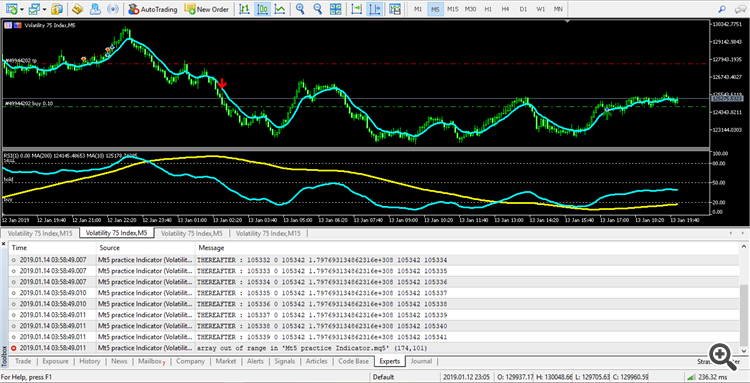Error Line:

Files: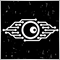45361

Harrysung:

Hello guys;

I have an issue, I am trying to code an Arrow indicator for Moving Average crossover, but I always encounter an "Array out of Range Error" on any line with [i+2], and when i cooment out this lines, I later have the same "Array out of Range Error" on any line with [i+1] && [i+3]. I have been trying to rectify this issue for hours but have been going nowhere. This is very important to me as i am trying to port my self written Indicators from Mt4 to Mt5.

Below is the Error ScreenShot:

try:

for(int i=(int)MathMax(prev_calculated-1,3); i<rates_total; i++) {22

Minions Labs:

try:

I tried it : still  "Array Out Of Range Error".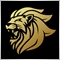Moderator
12472

You should check the arraysize with the location you are either trying to write to or to read from because at some point it's none existent.

Now it tells you the problem is at line 176, cursor position 101, but we do not see line numbers so we can not check which line that is.22

Marco vd Heijden:

You should check the arraysize with the location you are either trying to write to or to read from because at some point it's none existent.

Now it tells you the problem is at line 176, cursor position 101, but we do not see line numbers so we can not check which line that is.

I don't quite get what you mean, but i have provided a picture of the error line and attached the file.Moderator
12472

What part do you not understand ?

When you check if the array location exists, before reading it you can prevent trying to read a non existent location.22

Marco vd Heijden:

What part do you not understand ?

When you check if the array location exists, before reading it you can prevent trying to read a non existent location.

How can I check if location exists please? I tried checking for array size up to that error point and the size was what it should be.2956

Array MA[] contains rates_total elements indexed from 0 to rates_total-1.

Your loop index variable "i" iterates up to rates_total-1.

When i == rates_total-1, you try to read MA[i+1] and MA[i+2]

i+1 = rates_total-1+1 = rates_total

i+2 = rates_total-1+2 = rates_total+1

This causes "Array out of range error" because the last index in the MA[] array is rates_total-1Moderator
12472

Harrysung:

How can I check if location exists please? I tried checking for array size up to that error point and the size was what it should be.

If the position you are going to read is bigger then the size of the array....

You could do a simple if([i+2]<0) {i=0;}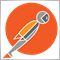2391

The true issue resides in the logic of your for loop.

for(int i=(int)MathMax(prev_calculated-1,1); i<rates_total; i++) {

So lets say for argument sake that rates_total = 1000. When prev_calculated also equals 1000, the loop starts off at index=999, which is exactly the last element in the array. Therefore, any attempt to increment the beginning index beyond 999 will result in an index out of range error. If you need to increment the index in the body of the for loop block then you will need to account for that in the loop expression.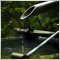1888

Change that loop to this will do the trick.

for(int i=(int)MathMax(prev_calculated-1,1); i<rates_total && i+2<ArraySize(MA2); i++)